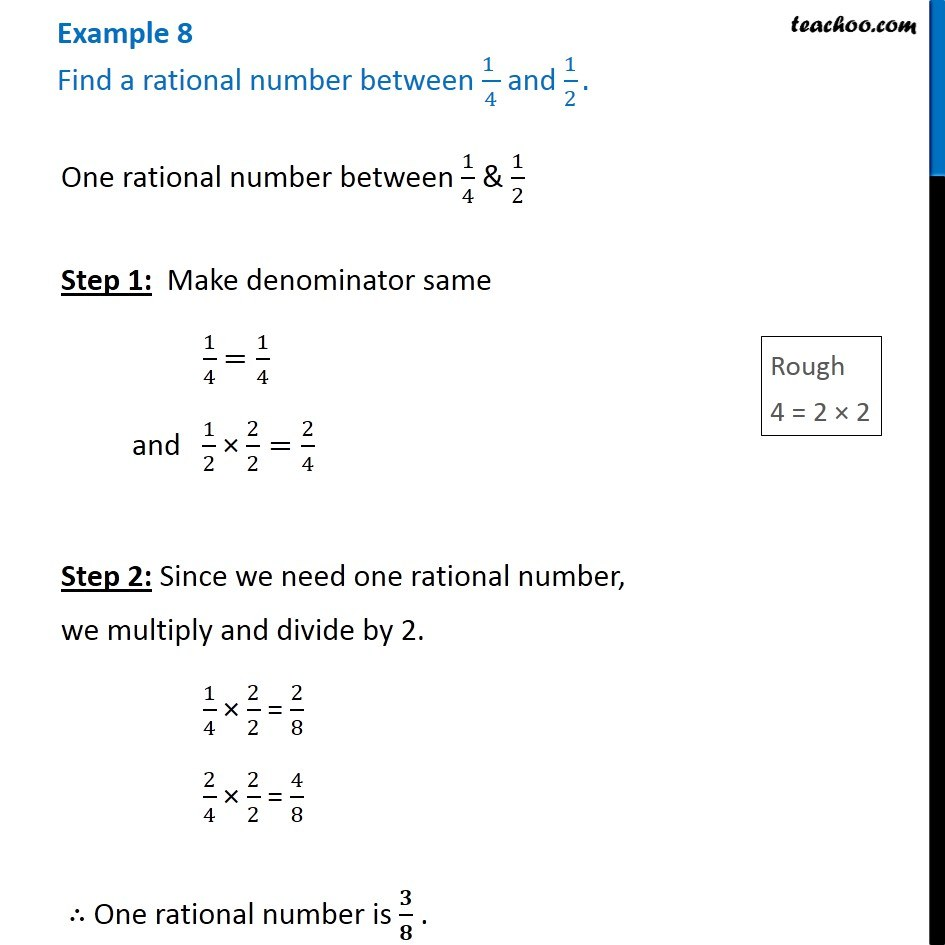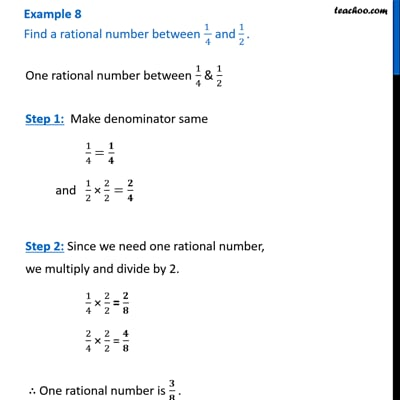Examples

Chapter 1 Class 8 Rational Numbers
Serial order wiseThis video is only available for Teachoo black users

Get live Maths 1-on-1 Classs - Class 6 to 12

### Transcript

Example 8 Find a rational number between (1 )/4 and 1/2. One rational number between 1/4 & 1/2 Step 1: Make denominator same 1/4 =1/4 and 1/2 × 2/2=2/4 Step 2: Since we need one rational number, we multiply and divide by 2. 1/4 × 2/2 = 2/8 2/4 × 2/2 = 4/8 ∴ One rational number is 𝟑/𝟖 .